# Describing functions¶

For nonlinear systems consisting of a feedback connection between a linear system and a static nonlinearity, it is possible to obtain a generalization of Nyquist’s stability criterion based on the idea of describing functions. The basic concept involves approximating the response of a static nonlinearity to an input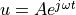as an output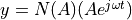, where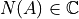represents the (amplitude-dependent) gain and phase associated with the nonlinearity.

Stability analysis of a linear system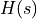with a feedback nonlinearity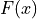is done by looking for amplitudes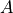and frequencies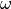such that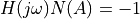If such an intersection exists, it indicates that there may be a limit cycle of amplitudewith frequency.

Describing function analysis is a simple method, but it is approximate because it assumes that higher harmonics can be neglected.

## Module usage¶

The function describing_function() can be used to compute the describing function of a nonlinear function:

N = ct.describing_function(F, A)


Stability analysis using describing functions is done by looking for amplitudes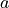and frequencies :mathomega such that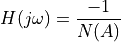These points can be determined by generating a Nyquist plot in which the transfer function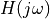intersections the negative reciprocal of the describing function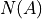. The describing_function_plot() function generates this plot and returns the amplitude and frequency of any points of intersection:

ct.describing_function_plot(H, F, amp_range[, omega_range])


## Pre-defined nonlinearities¶

To facilitate the use of common describing functions, the following nonlinearity constructors are predefined:

friction_backlash_nonlinearity(b)     # backlash nonlinearity with width b
relay_hysteresis_nonlinearity(b, c)   # relay output of amplitude b with
# hysteresis of half-width c
saturation_nonlinearity(ub[, lb])     # saturation nonlinearity with upper
# bound and (optional) lower bound


Calling these functions will create an object F that can be used for describing function analysis. For example, to create a saturation nonlinearity:

F = ct.saturation_nonlinearity(1)


These functions use the DescribingFunctionNonlinearity, which allows an analytical description of the describing function.

## Module classes and functions¶

 Base class for nonlinear systems with a describing function. Backlash nonlinearity for describing function analysis. Relay w/ hysteresis nonlinearity for describing function analysis. saturation_nonlinearity([ub, lb]) Create saturation nonlinearity for use in describing function analysis.